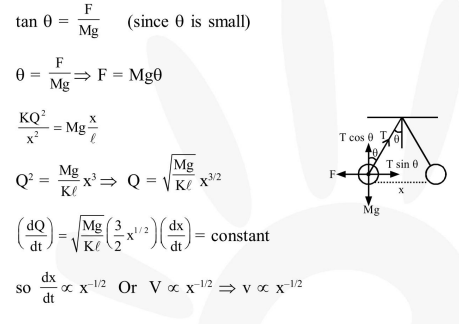# Two identical charged spheres suspended from a common point by two massless string of length

Question:

Two identical charged spheres suspended from a common point by two massless string of length $\ell$ are initially a distance $\mathrm{d}(\mathrm{d} \ll \ell)$ apart because of their mutual repulsion. The charge begins to leak from both the spheres at a constant rate. As a result the charges approach each other with a velocity v. Then as a function of distance $x$ between them :-

1. $\mathrm{V} \propto \mathrm{X}^{1 / 2}$

2. $V \propto x$

3. $\mathrm{V} \propto \mathrm{x}^{-1 / 2}$

4. $v \propto x^{-1}$

Correct Option: , 3

Solution: